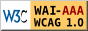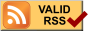Octave note

簡介

GNU Octave 是一種科學計算軟體，它提供了方便的互動命令列介面來解決線性與非線性的數值運算問題，並可將計算結果可視化，與高價的商業軟體MATLAB語法幾乎相容。在此推薦教授「科學計算」的老師們不要教 MATLAB而改教Octave或Scilab，畢竟教學是教方法與概念而非教哪一間公司的商業軟體，除了MATLAB現有的程式檔幾乎可無誤的在Octave或Scilab上跑之外，也建立學生們尊重智財權與學習自由軟體的概念。

Octave使用GPL授權，類似的Matlab clone軟體還有 Scilab, Rlab 等。 Octave的繪圖功能是利用gnuplot達成的，不像MATLAB 繪圖是內建功能，在安裝Octave時會一併安裝其使用手冊與gnuplot。

Octave的命令模式可使用的按鍵操作與emacs相同，像是Ctrl+U, Ctrl+K, Ctrl+A, Ctrl+E, .......等。

Octave是一個運算式(expression)的語言，整個Octave程式可看成對運算式的解釋及計算，常見的形式有

1. variable=expression
octave:1> a= [1 2; 3 4] ; % 運算式最後的分號有抑制結果顯示的作用
octave:2 > b= [2 3; 4 5] ; % 方括弧代表矩陣，並以分號代表不同row
octave:3 > c=a+b
c =
3      5
7      9
octave:4>
2. expression 或 variable
octave:4> (8+3) * 5 % 運算結果會存到預設變數 ans
ans = 55
octave:5> z = 10*sin(pi/3)*...
sin(pi/3) ; % 利用三個句點「...」可將過長的運算式拆成二行
octave:6> z % 變數名可直接顯示變數結果，而無須前置動詞 disp
z = 7.5000

變數(Variable)

• 變數的第1個字必須是英文或底線，不可為數字，可利用isvarname函數測試命名的變數是否成立。
octave:1> isvarname 3abc
ans = 0
octave:2> isvarname _abc
ans = 1
octave:3> isvarname abc3_
ans = 1
• 變數無須宣告(variable declaration)即可使用，預設以double(8 bytes)資料型態儲存。
• 不限制變數名稱長度。(MATLAB預設為63個字元)
• 大小寫不同視為不同的變數。
octave:4> a=1 ; A=3 ; a+A
ans = 4
• 避免使用函數名稱來當變數名稱，因為這樣會變成變數可使用，但同名稱的函數無法使用了。
octave:5> disp "HelloWorld" % 正常的disp 函數功能
HelloWorld
octave: 6>  disp = 50 ;  % 誤將函數名稱當變數名稱使用後
octave: 7> disp "HelloWorld"  % 造成disp函數呼叫後產生下列錯誤訊息
error: octave_char_matrix_str::valid_as_scalar_index() : not implemented
octave:8> clear
• 使用「which 名稱」，可得知此名稱是變數名稱還是函數名稱。
octave:9> s = [1 3 5 7] ;
octave:10> which s
s is a user-defined variable
octave:11 > which clear
clear is a built-in command
• 可使用whowhos指令顯示現存工作空間(workspace)的所有變數資訊。
• 使用clear 可將現有記憶體中的所有變數清除。

MATLAB

• MATLAB的變數規則大都如上述，惟變數名稱長度會根據namelengthmax函數傳回的數值決定，目前預設為63，超過的部分忽略不管，但有警告訊息。
>>> namelengthmax
ans =
63
>>> abcdefghijklmnopqrstuvwxyzabcdefghijklmnopqrstuvwxyzabcdefghijklmnopqrstuvwxyz = 10
Warning: 'abcdefghijklmnopqrstuvwxyzabcdefghijklmnopqrstuvwxyzabcdefghijklmnopqrstuvwxyz' exceeds MATLAB's maximum name length of 63 characters and has been truncated to 'abcdefghijklmnopqrstuvwxyzabcdefghijklmnopqrstuvwxyzabcdefghijk'.
(Type "warning off MATLAB:namelengthmaxexceeded" to suppress this warning.)
abcdefghijklmnopqrstuvwxyzabcdefghijklmnopqrstuvwxyzabcdefghijk =
10

矩陣(Matrix)

• 矩陣資料是Octave最基本且重要的資料形式，其中1x1階的矩陣資料稱之為純量(scalar)，而一列(row)或一行(column)的矩陣資料稱之為向量(vector)。
• 矩陣資料的構成如下
1. 矩陣由方括弧構成，如 array=[1 2 3]
2. 矩陣元素可以為任何的expression，其間以空白或逗點隔開，如 array=[-2.5,  sqrt(4^2+5),  2*exp(i*pi/3)]
3. 矩陣的每個row以分號 ; 結束，如 array=[1 2 3; 4 5 6; 7 8 9]
• 矩陣中的元素指定方式為 「矩陣名稱(駐標)」，例如 array(2,3) =25
• Octave的矩陣無須宣告就能使用，但可使用 zeros, ones, rand, randn 等函數來事先定義大小與初值，如 array= 5*ones(3, 2)
• 多維矩陣可利用 cell, zeros, ones, rand, randn 等函數來產生之，其中cell的陣列元素亦是由陣列構成

網路資源|||||